# Easy Guide – How to Calculate Voltage Drop Across Resistors

• GuidesUnderstanding how to calculate the voltage drop is part of the foundational knowledge for people interested in creating their own electronic circuits. Finding the voltage drop can be confusing as calculation methods can change depending on the circuit and the number of resistors within the circuit, which we will explain in this article.

Before we jump in straight into how to calculate the voltage drop across resistors, we are going to look at basic knowledge useful not only for calculating the voltage drop across a resistor but also for developing any kind of electronic circuit.

## What is the voltage drop across a resistor?

Talking about calculating the voltage drop across a resistor wouldn’t make sense if we don’t understand what is the voltage drop across a resistor. Let’s take the following circuits in the image below to help provide more clarity.

In the image above, you will see two circuits. The first circuit with only one resistor, and the second circuit with two resistors. When talking about the voltage drop across a resistor, we are referring to the voltage across a resistor, or the voltage once the current flow has gone through a resistor.

## Undestanding the Ohm’s Law

If you haven’t heard of Ohm’s law before, we are going to take a quick stop to understand it.

Ohm’s law is a formula commonly taught to students of electronics and it helps to calculate the relationship between the voltage, current, and resistance of a circuit. Ohm’s law is best described by using a pyramid displaying the relationship between these three values.

Ohm’s law states that if there are two known values in a circuit, such as the current and the resistance, we can find the third value by modifying the position of the values in the pyramid and doing a simple calculation.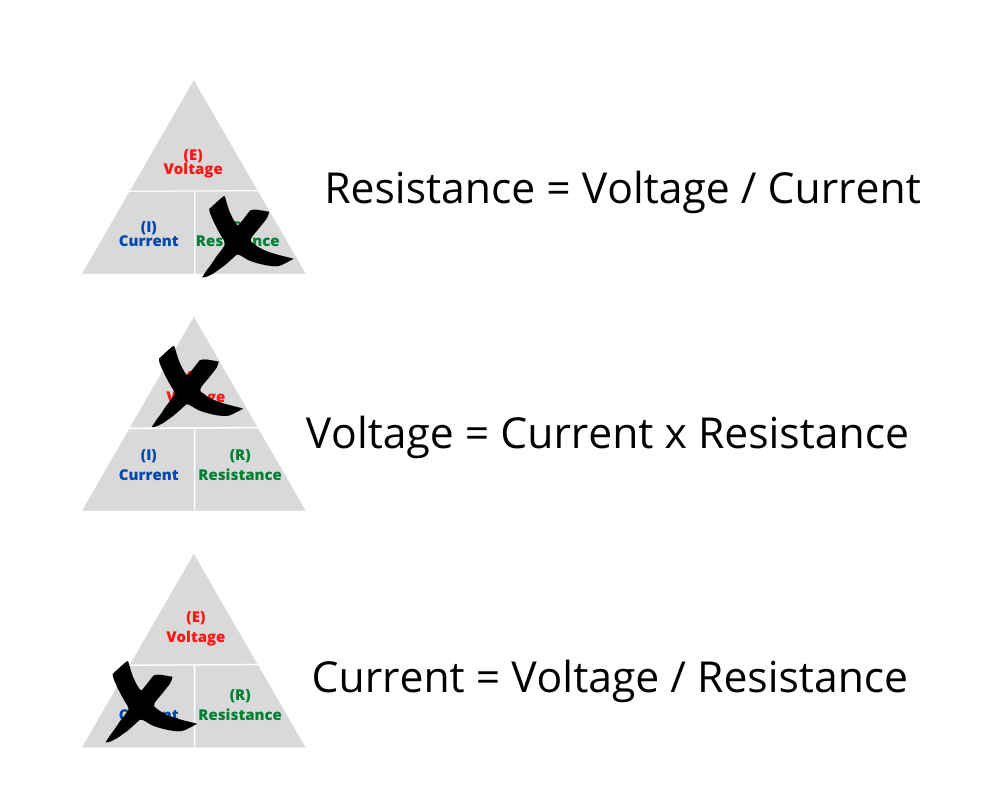Formulas to calculate Voltage, Current, and Resistance using Ohm’s Law

In this way, we can have the following formulas:

• Resistance is equal to the voltage divided by the current
• Voltage is equal to the current times the resistance value
• Current is equal to the voltage devided by the resistance value

## Calculate Voltage Drop Across Resistors Based on the Circuit Type

### Series Circuit

#### What is a Series Circuit?

A series circuit is a circuit where the current flows in only one direction through each component. In the following example, notice how there is one path and how the current has to flow across the two resistances, R1 and R2.

Series circuits have one principle which we will need to take into account when calculating the voltage drop across resistors: the current is the same through all of the components of the circuit. If we apply this concept to the previous circuit, that means the current will be the same after resistance R1 and resistance R2.

#### How do you calculate the current in a series circuit?

Unfortunately, we don’t know the current that flows in the whole circuit in our previous example.

Do you remember the ohm’s law formula we covered earlier?

By rearranging the elements correctly from the ohm’s law pyramid, we can calculate the current using the voltage of the series circuit divided by the resistance.

We know the voltage (V1) of the circuit is 9V. However, we don’t know the total resistance of the circuit, even though we know all the resistance values of all the resistors of a circuit.

How do you determine the total resistance value of the series circuit?

This leads us to another principle of series circuits: The total resistance of a circuit is equal to the sum of all the individual resistances.

That means we can do a simple math calculation to get the total resistance of the circuit:

Resistance = 220Ω + 220Ω

Resistance = 440Ω

Knowing the resistance and the voltage of the circuit, we can now calculate the current using ohm’s law:

Current = 9V / 440Ω

Current = 0.2A

#### How to calculate voltage drop?

At this point, you might wonder why we are calculating the current of the circuit when what are looking for is to calculate the voltage drop across the resistors of the circuit.

To calculate the voltage drop of a resistor in a series circuit, we are going to use ohm’s law which states that the voltage is equal to the current times the resistance value.

We are going to see a few examples to calculate the voltage drop. However, before checking at some examples, there is another principle that serves as guide to determine if the calculations are correct: the total voltage of a series circuit is equal to the sum of all of the individual voltages.

##### Example 1

In this first example, we are going to continue with the original diagram we have been using to explain what the series circuit is and its related principles.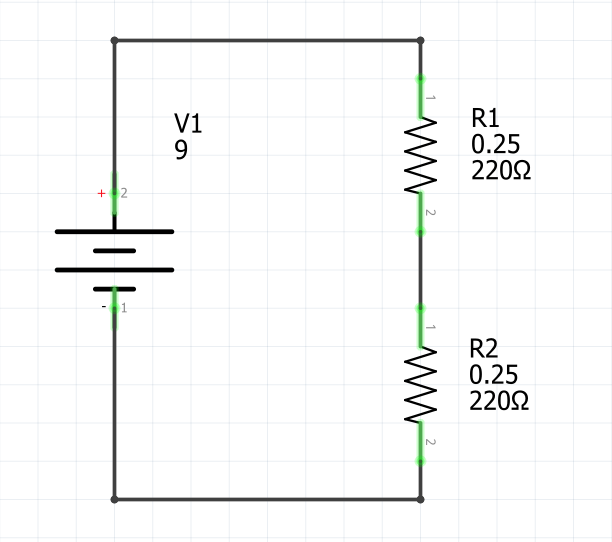Example #1: Calculate voltage drop of each resistor in a series circuit

Given the following values:

• Total Voltage = 9V
• Resistance R1 = 220Ω
• Resistance R2 = 220Ω
• Current = 0.02A

Find the voltage drop of resistor R1 and resistor R2.

Solution

Voltage for Resistor R1 = 0.02A x 220Ω

Voltage for Resistor R1 = 4.5V

Since the resistance value of resistor R2 is the same, we can infer the voltage drop for resistor R2 is the same as the voltage drop calculated for resistor R1.

Voltage for Resistor R2 = 0.02A x 220Ω

Voltage for Resistor R2 = 4.5V

In case we need to verify the voltage drop across each resistor, we can take as a reference the principle of summing all the individual voltages of the circuit should be equal to the voltage of the series circuit.

Total Voltage = Voltage R1 + Voltage R2

Total Voltage = 9V = 4.5V + 4.5V

##### Example 2

Let’s look at a much simpler series circuit example.

Given the following values:

• Voltage = 12V
• Resistance R1 = 330Ω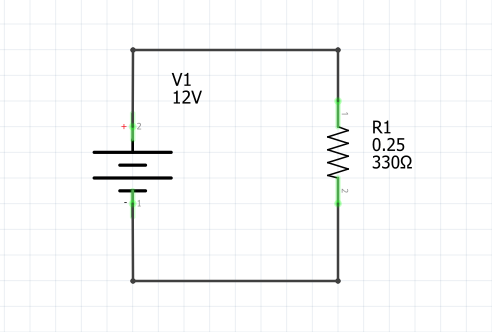Example #2: Calculate voltage drop of each resistor in a series circuit

Find the voltage drop across the resistor R1 and the current that flows across resistor R1.

Solution

In this case, we don’t need to calculate the voltage drop across resistor R1 as the exercise is already telling us the voltage. By using the principle of summing all the individual voltages of the circuit should be equal to the voltage of the series circuit, we can infer that voltage of resistor R1 is 12V.

Total Voltage = Voltage R1

12V = 12V

To calculate the current that flows across resistor R1, we are going to find the current of the whole circuit.

Remember the first principle we covered in the series circuit?

The current is the same through all of the components of the circuit. Hence we are going to use the ohm’s law formula as we already have the voltage across the resistor R1 and its resistance value.

Current = 12V / 330Ω

Current = 0.036A

##### Example 3

Let’s look at an example with more resistors components in the circuit.

Given the following values:

• Voltage = 5V
• Resistance R1 = 33Ω
• Resistance R2 = 100Ω
• Resistance R3 = 4.7kΩ
• Resistance R4 = 220Ω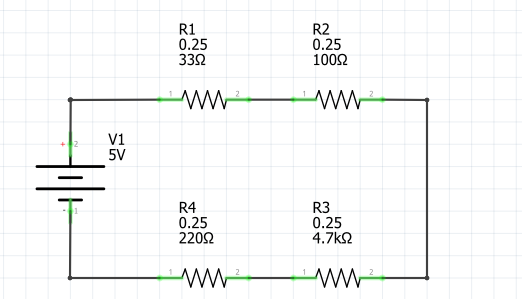Example #3: Calculate voltage drop of each resistor in a series circuit

Find the voltage drop across the resistors R1, R2, R3, and R4, and the current that flows across the whole circuit.

Solution

To calculate the current of the circuit we are going to use the ohm’s law formula as we need to get the total resistance value of the circuit.

Total Resistance = R1 + R2 + R3 + R4

Total Resistance = 33Ω + 100Ω + 4.7kΩ + 220Ω

Total Resistance = 5,053Ω

Now we can find the total current since we have the total voltage and the total resistance.

Total Current = Total Voltage / Total Resistance

Total Current = 5V / 5,053Ω

Total Current = 0.00098A

Now that have the total current of the circuit, that means we have the current that flows across all resistors.

Current Resistor R1 = 0.00098A

Current Resistor R2 = 0.00098A

Current Resistor R3 = 0.00098A

Current Resistor R4 = 0.00098A

That means we can calculate the voltage drop or simply the voltage across each resistor as we have the resitance value as well as the current value.

Voltage for Resistor R1 = 0.00098A x 33Ω = 0.032V

Voltage for Resistor R2 = 0.00098A x 100Ω = 0.098V

Voltage for Resistor R3 = 0.00098A x 4.7kΩ = 4.6V

Voltage for Resistor R4 = 0.00098A x 220Ω = 0.21V

In case we need to verify the voltage drop across each resistor, we can take as a reference the principle of summing all the individual voltages of the circuit should be equal to the voltage of the series circuit.

Total Voltage = Voltage R1 + Voltage R2 + Voltage R3 + Voltage R4

Total Voltage = 5V ≈ 0.032V + 0.098V + 4.6V + 0.21

### Parallel Circuit

#### What is a Parallel Circuit?

A parallel circuit is a circuit that where the electricity can travel across several different paths. A common analogy used to think of parallel circuit is a river that is divided into several different streams.

Parallel circuits are different from series circuit as the current value can be different on each path it travels.

#### How to calculate voltage drop?

If you look at the previous parallel circuit diagram, we have two different paths were the current flows. This technichally means there are two series circuits in case each resistor (R1 and R2) would not be connected in the circuit using the same voltage.

If you remember all the series circuit principles, one states that the total voltage of a series circuit is equal to the sum of all of the individual voltages.

Knowing that, we can determine the voltage drop without doing any calculations for resistors R1 and R2 as we know the total voltage of the circuit.

Voltage Resistor R1 = 9V

Voltage Resistor R2 = 9V

In this case, the voltage is the same in both paths of the parallel circuit. However, the current can be different on each path.

Current Resistor R1 = Voltage Resistor R1 / Resistance R1

Current Resistor R1 = 9V / 330Ω = 0.027A

Current Resistor R2 = Voltage Resistor R2 / Resistance R2

Current Resistor R2 = 9V / 100Ω = 0.09A

Let’s look at a few examples to calculate the voltage drop in different parallel circuits.

##### Example 1

Given the following circuit.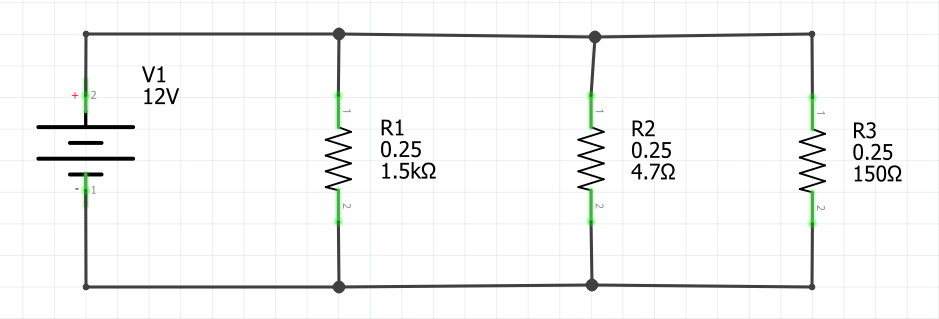Example #1: Calculate voltage drop of each resistor in a series circuit

Calculate the voltage drop across resistors R1, R2, and R3.

Solution

All of the paths in the parallel circuit have only one resistor in serie. This is easier to visualize if we split the parallel circuit into multiple series circuit, we will have the following circuits.

That means, the voltage across each resistor is the same as the total voltage of the circuit.

Voltage Resistor R1 = 12V

Voltage Resistor R2 = 12V

Voltage Resistor R3 = 12V

##### Example 2

Let’s look at a little more complex example. Given the following circuit.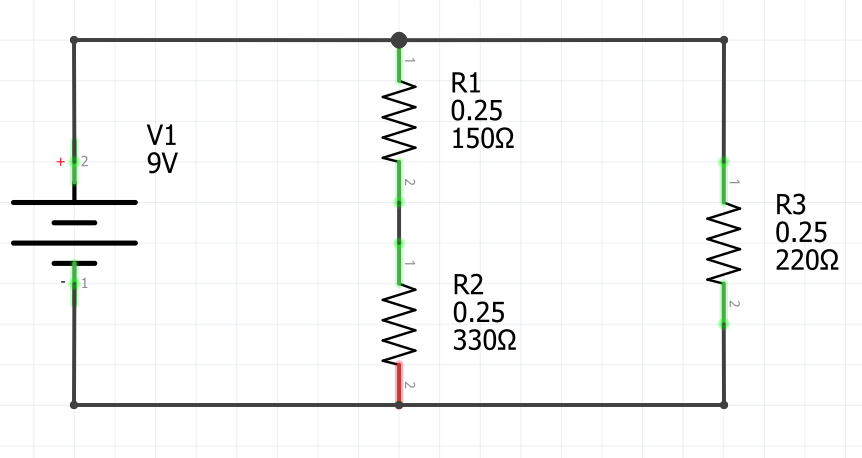Example #2: Calculate voltage drop of each resistor in a series circuit

Calculate the voltage drop across resistors R1, R2, and R3.

Solution

To make things simple, I recommend splitting the parallel circuit into multiple series circuits.

In this way, we can infer the voltage across resistors R3 will be the same of the total voltage of the circuit.

Voltage Resistor R3 = 9V

This is a little different in the case of the other series circuit, we first need calculate the total current flowing that circuit so we can apply ohm’s law formula to calculate the voltage across resistors R1 and R2.

Remember, the current is the same through all of the components in a series circuit. Hence, we will sum all resistors in the circuit and calculate the voltage.

Total Current in Series Circuit = 9V / (150Ω + 330Ω) = 0.01875A

Total Current in Series Circuit = Current Resistor R1 = Current Resistor R2

Current Resistor R1 = 0.01875A

Current Resistor R2 = 0.01875A

Now, that we have the current flowing on each resistor (R1 and R2), we can calculate the voltage drop across the resistors.

Voltage for Resistor R1 = 0.01875A x 150Ω = 2.8125V

Voltage for Resistor R2 = 0.01875A x 330Ω = 6.1875V

## Why do I need to drop the voltage in a circuit?

The best way to understand why we need to drop the voltage in a circuit is by looking at an example. If you have an LED light bulb and 9V battery, Would you connect to LED light directly to the battery?

It depends.

We need to look at the acceptable voltage range the LED light needs to turn on the light. Generally, LED lights have a voltage range between 1.8V to 3.4V depending on the color of the LED.

Now, if we know the voltage range for our LED light is between 3V and 3.2V, and we connect the LED directly to the battery, it will kill the LED.

Hence, we need to calculate the how much resistance we can use to drop the voltage to the 9V battery. Once we do the calculation, we find apropriate resistor using the resistor color code to determine the correct one. Finally, we use the resistor in the circuit in series with the LED light, preventing from killing the light bulb once the battery is connected.

## Conclusion

Calculating the voltage drop across resistors can be confusing at first. Tips to make this calculation simpler are:

• Understand the ohm’s law and it’s different variations to the formula to find resistance, voltage, and current on a circuit and components of the circuit.
• Determining what kind of circuit we have, whether it is a series circuit or a parallel circuit
• Understanding the principles of series and parallel circuits
• Convert a parallel circuit into multiple series circuits.